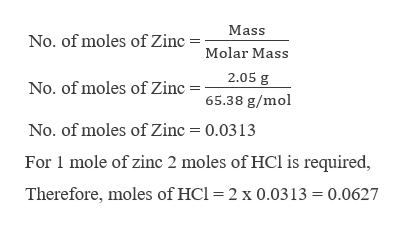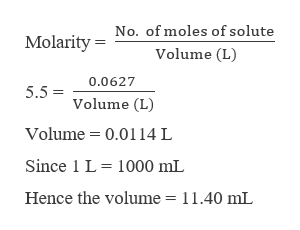# Zinc reacts with hydrochloric acid according to the reaction equation Zn(s)+2HCl(aq)⟶ZnCl2(aq)+H2(g)Zn(s)+2HCl(aq)⟶ZnCl2(aq)+H2(g) How many milliliters of 5.50 M HCl(aq)5.50 M HCl(aq) are required to react with 2.05 g Zn(s)?

Question

Zinc reacts with hydrochloric acid according to the reaction equation

Zn(s)+2HCl(aq)⟶ZnCl2(aq)+H2(g)Zn(s)+2HCl(aq)⟶ZnCl2(aq)+H2(g)

How many milliliters of 5.50 M HCl(aq)5.50 M HCl(aq) are required to react with 2.05 g Zn(s)?

check_circleExpert Solution
Step 1

The reaction of zinc with hydrochloric acid is shown as,

Step 2

It is required to calculate the volume in mL of 5.50 M HCl which will react with 2.05 g of Zinc. To calculate the volume, first the number of moles of HCl must be calculated, which can be done as,help_outlineImage TranscriptioncloseMass No. of moles off Zinc =Molar Mass 2.05 g No. of moles of Zinc 65.38 g/mol No. of moles of Zinc 0.0313 For 1 mole of zinc 2 moles of HCl is required, Therefore, moles of HCl = 2 x 0.0313 0.0627 fullscreen
Step 3

Now the volume of 5.5 M HCl ...help_outlineImage TranscriptioncloseMolarity=No. of moles of solute Volume (L) 0.0627 5.5 Volume (L) Volume 0.0114 L Since 1 L 1000 mL Hence the volume = 11.40 mL fullscreen

### Want to see the full answer?

See Solution

#### Want to see this answer and more?

Solutions are written by subject experts who are available 24/7. Questions are typically answered within 1 hour*

See Solution
*Response times may vary by subject and question
Tagged in

### Chemistry